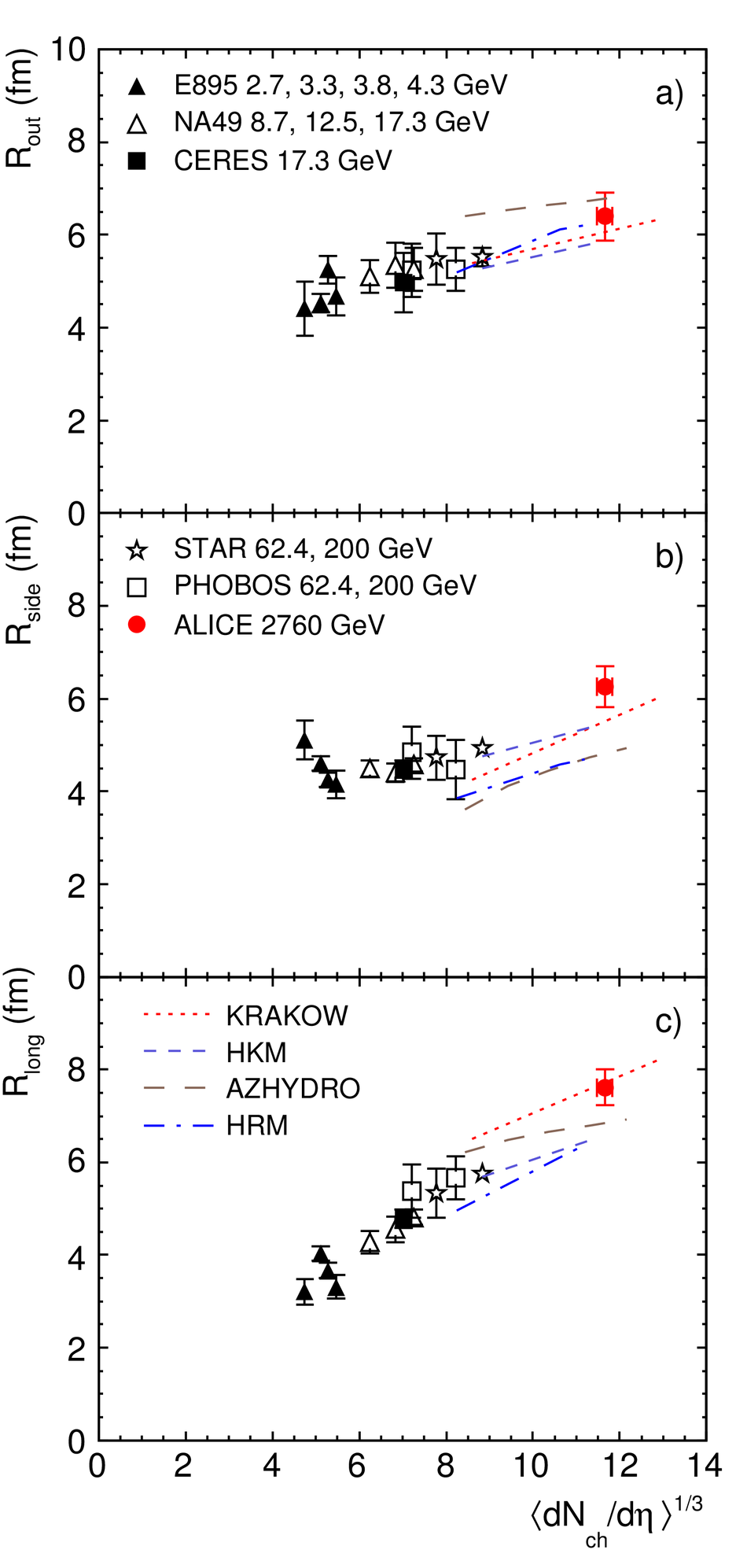# Two-pion Bose-Einstein correlations in central Pb-Pb collisions at $\sqrt{s_{\rm NN}}$ = 2.76 TeV

The first measurement of two-pion Bose--Einstein correlations in central Pb-Pb collisions at  $\sqrt{s_{\rm NN}}$ = 2.76 TeV at the Large Hadron Collider is presented. We observe a growing trend with energy now not only for the longitudinal and the outward but also for the sideward pion source radius. The pion homogeneity volume and the decoupling time are significantly larger than those measured at RHIC.

Figures

## Figure 3

 Pion HBT radii at $k_T$ = 0.3 GeV/$c$ for the 5% most central Pb-Pb at $\sqrt{s_{\rm NN}}=2.76~$TeV(red filled dot) and the radii obtained for central gold and lead collisions at lower energies at the AGS , SPS [36,37,38] and RHIC [39,40,41,42,30,43]. Model predictions are shown as lines.## Figure 4

 Product of the three pion HBT radii at $k_T$ = 0.3 GeV/$c$ The ALICE result (red filled dot) is compared to those obtained for central gold and lead collisions at lower energies at the AGS , SPS [36,37,38] and RHIC [39,40,41,42,30,43].## Figure 5

 The decoupling time extracted from $R_{\rm long}$($k_T$). The ALICE result (red filled dot) is compared to those obtained for central gold and lead collisions at lower energies at the AGS , SPS [36,37,38] and RHIC [39,40,41,42,30,43].